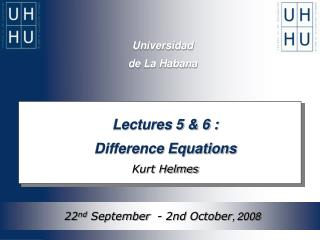# Universidad de La Habana - PowerPoint PPT PresentationDownload PresentationDownload Presentation- - - - - - - - - - - - - - - - - - - - - - - - - - - E N D - - - - - - - - - - - - - - - - - - - - - - - - - - -
##### Presentation Transcript

1. Universidad de La Habana Lectures 5 & 6 : Difference Equations Kurt Helmes 22nd September  - 2nd October,2008

2. CONTENT Part 1: Introduction Part 2: First-Order Difference Equations Part 3: First-Order Linear Difference Equations

3. 1 Introduction Difference Equations (Prof. Dr. K. Helmes)

4. Part 1.1 An Example Difference Equations (Prof. Dr. K. Helmes)

5. Example 1 (Part 1) cf. compound interest Dagobert- Example

6. interest factor Starting Point: Given: K0 initial capital ( in Euro ) p interest rate ( in % ) r

7. Objective: Find .... • 1. The amount of capital after 1 year. • 2. The amount of capital after 2 years. • n. The amount of capital after n years.

8. Solution: After oneyear the amount of capital is: How much capital do we have after 2 years?

9. Solution: After one year the amount of capital is: After twoyears the amount of capital is:

10. Solution: After one year the amount of capital is: After twoyears the amount of capital is:

11. Solution: After nyears the amount of capital is:

12. The solution formula can be rewritten in the following way: is given, is given, Observation: special difference equation recursion formula

13. Part 1.2 Difference Equations Difference Equations (Prof. Dr. K. Helmes)

14. Illustration: A difference equation is a special system of equations, with • (countably) infinite many equations, • (countably) infinite many unknowns.

15. Hint: The solution of a difference equation is a sequence (countably infinite many numbers).

16. How do we recognize a difference equation?

17. An equation, that relates for any the nthterm of a sequence to the (up to k)preceding terms, is called a (nonlinear) difference equation of order k. Definition: Difference Equation Explicit form: Implicit form:

18. 2 First-Order Difference Equations Difference Equations (Prof. Dr. K. Helmes)

19. Part 2.1 A Model for the„Hog Cycle“ Difference Equations (Prof. Dr. K. Helmes)

20. Example 2 cf. Microeconomic Theory „Hog Cycle“ (Example)

21. ratio 16 12 Avg 8 year Starting Point: Given: Hog-corn price ratio in Chicago in the period 1901-1935:

22. price ratio time Starting Point: Stylized:

23. Starting Point: • Find: • A (first) model, which „explains“ / describes the cyclical fluctuations of the prices (ratio of prices).

24. in units at time in units at time in units at time in units at time Model (Part 1): Supply and Demand The suppply of hogs: The demand of hogs:

25. The supply at time depends on the hog price at time . Model (Part 2): Supply and Price Assumption:

26. i.e. it is determined by and , and p(t). is given Model (Part 2): Nature of the dependance Assumption: The supply function is linear:

27. parameter Model (Part 3): Demand and Price Assumption: For the demand we assume: If the hog price increases, the demand will decrease, thus:

28. for all Model (Part 4): Equilibrium Postulate: Supply equals demand at any time:

29. Model (Part 4): Equilibrium The equilibrium relation yields a defining equation for the price function:

30. is given Solution (Part 4): Equilibrium Thus we obtain the following difference equation:

31. Model (Part 4): Equilibrium This difference equation is: • first-order • linear • inhomogeneous

32. Model (Part 5): Analysis solution formula:

33. a = : 1 e.g. b = 3 g = 2 d = 5 Iteration rule Figure 2:

34. „stable“: The values converge to the equilibrium state when . Model (Part 5): Analysis Results: The equation / solution is stable. The equation / solution is unstable.

35. 1 0,8 0,6 0,4 0,2 0 -0,2 -0,4 0 10 20 30 40 5 15 25 35 Figure 3:Price development for:

36. 1 0,8 0,6 0,4 0,2 0 -0,2 -0,4 16 0 4 10 14 20 2 6 8 12 18 Figure 4:Price development for:

37. 40 30 20 10 0 -10 -20 -30 0 10 20 30 40 5 15 25 35 Figure 5:Price development for:

38. The term has an alternating sign, . Summary: The given difference equation has a unique solution; it can be solvedexplicitly. The price is the sum of a constant and a power function.

39. CONCLUSION: We can model and analyze dynamic processeswith difference equations.

40. Part 2.2 Definitions und Concepts for First-Order Difference Equations Multivariable Calculus: The Implicit Function Theorem (Prof. Dr. K. Helmes)

41. Definition: A (general) first-order nonlinear difference equation has the form : (F is defined for all values of the variables.)

42. Important Questions: • Does at least one solution exist? • Is there a unique solution? • How many solutions do exist? • How does the solution change, if „parameters“ of the system of equations are changed (sensitivity analysis)?

43. Important Questions: • Do explicit formulae for the solution exist? • How do we calculate the solution? • Does the system of equations has a special structure ? e.g.: a) linear or nonlinear, b) one- or multidimensional ?

44. ”fixed number”, Remark: If the initial value of the solution (sequence) of a difference equation is given, i.e. then we call our problem an ” initial value problem ” related to a first-orderdifference equation.

45. If is an arbitrary fixed number, then there exists a uniquely determined function/sequence , that is a solution of the equation and has the given value for . Remark: The initial value problem of a first-order difference equation has a unique solution.

46. In general there exists for each choice of a different (corresponding) unique solution sequence. Remark:

47. For time homogeneous nonlinear difference equations we call points which satisfy the equation Definition: Invariant Points invariant points. F ”right-hand side”.